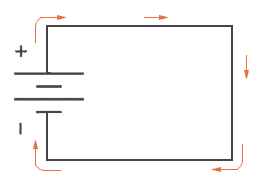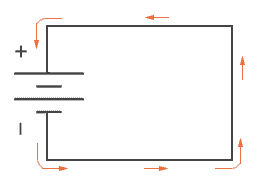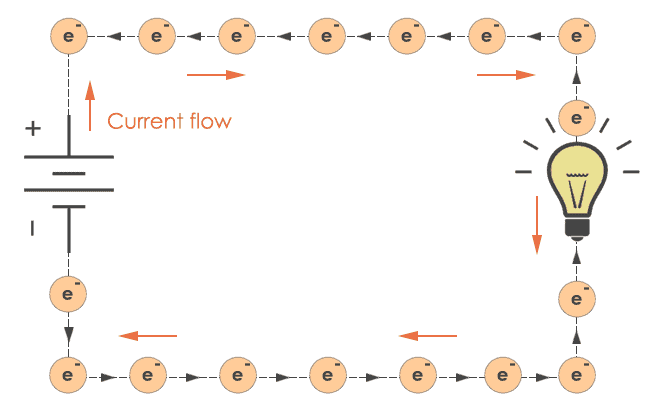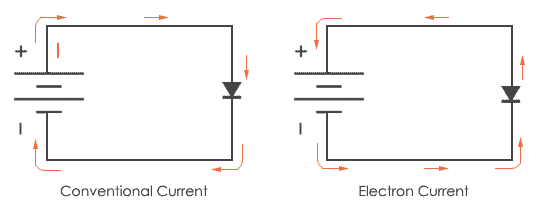# Conventional Current vs. Electron Current

Electron current and conventional current are two types of notation we use to mention current flow in a circuit. These two notations are opposite to each other. We need a notation to do some calculations like in Kirchhoff’s law. And we consider conventional current as the standard notation of current flow. But, actually this notation is scientifically not correct. But still, all the laws follow this notation. So before we get into these notations, we need to understand charges in electricity.

## Charges – Positive and Negative Charges

First of all Franklin assumed an electric charge which moved in the opposite direction that it should actually be doing, so he called this electric charge “negative” which means deficiency of charges and so we can consider “positive” means surplus of electric charges.

After some time, true direction of flow of electrons was discovered and it was noticed that this labels of positive and negative is incorrect scientifically. By that time, the notation of positive and negative was extremely widespread and so no efforts were made by engineers to change the old notations. As a result of this, we are still using the same notations that were first assumed by Sir Benjamin Franklin. And this notation we use now is conventional current notation or normal current flow. Let’s discuss it in detail.

## Conventional current Flow Notation

Before starting with this, we associate the word “positive” with surplus of charges and “negative” with deficiency of charges. This has been a label since Franklin first assumed it.

Imagine a battery connected across the conductor. In the electrically stressed conductor, electric charges move from positive terminal to negative terminal of the battery. Positive terminal has surplus of electric charges and so these charges are attracted towards negative terminal of the battery where there is a deficiency of charges. This notation is used widely by the engineers and so it is said as conventional flow notation.## Electron flow notation

As the name itself says, this notation is based on the movement of electrons. This notation shows what actually happens inside an electrically stressed conductor. The negative terminal of battery has high density of electrons. This electron travels from the negative terminal of the battery where the density of electrons is high to the positive terminal where the density of electrons is less and that’s why they get attracted towards positive terminal of the battery. Hence this type of current is known as electron current.## Conventional flow notation vs. Electron flow notationThis figure shows the difference between conventional current and electron current. They are exactly opposite to each other.

Now, we know the truth that electron flow notation is scientifically correct flow notation though we use conventional current flow most of the time. It doesn’t matter which one you are using you can have correct calculations while applying Ohm’s law, Kirchhoff’s law and all other equations. Most of the electronic semiconductors are designed on the basis of conventional current. For example, take diode; it is like a valve which allows current to flow in only one direction. Here if higher potential is applied to positive terminal of the diode and lower potential to negative terminal of the diode, then diode will allow passing through the current because this arrangement will break the depletion layer of the diode.And if we reverse the connections, diode will not let the current pass through it.

After all this, you will understand what scientifically true doesn’t matter a lot. You need to just stick to one of it, but just remember that the electrons are moving the other direction.